﻿ A Computational Mechanism for Analysis of the Functional Dependence of Solar Energy Transmissivity on Collector’s Latitude Angle and Exposure Time

### A Computational Mechanism for Analysis of the Functional Dependence of Solar Energy Transmissivity o...

C. I. Nwoye, C. N. Mbah, N. I. Amalu, S. O. Nwakpa, E. C. Chinwuko, I. E. NwosuOPEN ACCESSPEER-REVIEWED

## A Computational Mechanism for Analysis of the Functional Dependence of Solar Energy Transmissivity on Collector’s Latitude Angle and Exposure Time

C. I. Nwoye1,, C. N. Mbah2, N. I. Amalu3, S. O. Nwakpa1, E. C. Chinwuko4, I. E. Nwosu5

1Department of Metallurgical and Materials Engineering, Nnamdi Azikiwe University, Awka, Nigeria

2Department of Metallurgical and Materials Engineering, Enugu State University of Science & Technology, Enugu, Nigeria

3Project Development Institute, Enugu

4Department of Industrial and Production Engineering, Nnamdi Azikiwe University, Awka, Nigeria

5Department of Environmental Technology, Federal University of Technology, Owerri, Nigeria

### Abstract

This paper presents a factorial analysis of energy transmissivity by solar collector based on the collector exposure time and latitude angle of its location. A two-factorial model was derived and validated for the predictive analysis. The model structure highlighted the dependency of solar energy transmissivity on the collector exposure time and latitude angle of its location. ζ = - 0.0021θ - 5 x 10-6ϑ + 0.9081. The validity of the derived model was rooted on the core model expression ζ - 0.9081 = - 0.0021θ - 5 x 10-6 ϑ where both sides of the expression are correspondingly approximately equal. Regression model was used to generate results of transmissivty, and its trend of distribution was compared with that from derived model as a way of verifying its validity relative to experimental results. The results of this verification translated into very close alignment of curves and significantly similar trend of data point’s distribution for experimental (ExD), derived model (MoD) and regression model-predicted (ReG) results. Evaluations from generated results indicated that transmissivity per unit exposure time of collector and latitude angle of its location as obtained from experiment, derived model & regression model were 1.0545 x 10-5, 1.0545 x 10-5 & 1.0909 x 10-5 (day)-1 and 0.0040, 0.0040 & 0.0042 deg.-1 respectively. Standard errors incurred in predicting transmissivity for each value of the solar collector exposure time & latitude angle considered as obtained from experiment, derived model & regression model were 0.0003, 0.0002 & 2.1422 x 10-5 % and 0.0001, 0.0005 & 2.8396 x10-5 % respectively. The maximum deviation of model-predicted transmissivity (from experimental results) was less than 0.03% which is insignificant. This implies a model operational confidence level above 99.9%.

### At a glance: Figures

123
Prev Next

• Nwoye, C. I., et al. "A Computational Mechanism for Analysis of the Functional Dependence of Solar Energy Transmissivity on Collector’s Latitude Angle and Exposure Time." American Journal of Computing Research Repository 3.1 (2015): 9-13.
• Nwoye, C. I. , Mbah, C. N. , Amalu, N. I. , Nwakpa, S. O. , Chinwuko, E. C. , & Nwosu, I. E. (2015). A Computational Mechanism for Analysis of the Functional Dependence of Solar Energy Transmissivity on Collector’s Latitude Angle and Exposure Time. American Journal of Computing Research Repository, 3(1), 9-13.
• Nwoye, C. I., C. N. Mbah, N. I. Amalu, S. O. Nwakpa, E. C. Chinwuko, and I. E. Nwosu. "A Computational Mechanism for Analysis of the Functional Dependence of Solar Energy Transmissivity on Collector’s Latitude Angle and Exposure Time." American Journal of Computing Research Repository 3, no. 1 (2015): 9-13.

 Import into BibTeX Import into EndNote Import into RefMan Import into RefWorks

### 1. Introduction

Energy has been widely recognized as the most important and unavoidable necessity for economic development and industrialization. Its availability has also been considered a driving force and principal factor on which economic growth and industrial development are hinged.

Derived energy from the sun has been reported  find applicability in the areas of heat and electricity generation for domestic and industrial use, depending on the sun’s radiation on the collector location, duration of the radiant inflow, environmental weather condition, solar distance (i.e the distance of the array from the sun), time of the day at which the sun’s radiant heat is being collected, angle of incidence (i.e the angle between the normal to the solar array and a light ray from the sun) and on the light transmission losses in cover glasses, cover adhesive as well as other optical elements. Research  has shown that the length of time and proportion of the amount of solar energy radiation reaching the earth vary from place to place as a result of the earth’s orientation and attenuation factors.

Intensive studies  on solar energy, its mode of transmission and conversion reveal that the most effective process of converting solar radiation to useful thermal energy is Heliothermal. This process operates with the principle of operation in solar devices such as solar cooker, solar still, solar water disinfection, solar power desalination etc. The most important component of heliothermal system is the flat plate collector.

The scientists  reported that glass can transmit up to about 90% of the incident short wave radiation and has therefore become the most commonly used material in solar collectors. Its transmittance to the long wave heat radiation (5 to 50) emitted by the absorber plate was found very negligible. The report indicated that the transmission properties of the glazing cover remains the factor on which the intensity of solar radiation striking the absorber plate of a flat plate collector depends. Results of similar work  carried out by different researchers revealed that transmissivity varies with geographic location due to variation of solar geometry with location. The researchers posited that the transmissivity of a glazing cover is very important in designing and evaluating the performance of solar energy conversion systems.

In a previous work , transmissivity was calculated from associated solar geometrical factors using parametric definitions and computations. However, no mathematical relationship placed transmissivity of the glazing surface (used for the solar collector) as a function of solar collector exposure time and latitude angle of its location. These factors affect transmissivity very significantly because each point on the earth surface makes a different angle of incident with radiations from the sun.

The aim of this work is to carry out a factorial analysis energy transmissivity by the solar collector based solar collector exposure time and latitude angle of its location. This is in furtherance of the previous work . A model will be derived and used for this analysis. Regression model will also be used to compare the data distribution trend of the derived model as a way of verifying its validity. The model is expected to typify the thermal performance of a prototype solar collector.

### 2. Solar Energy Formation and Radiation

The sun generates radiant energy by combination of hydrogen atoms to form helium. The energy released in this process directly supplies most of the natural energy systems on earth which can be utilized by conversion of the heat to electricity .

### 3. Materials and methods

The glazing surface of a solar flat plate collector was positioned at different latitude angles to permit different amount of incident radiations from the sun. Details of the experimental procedure and associated process conditions are as stated in the past report .

3.1. Model Formulation

Experimental data obtained from research work  were used for this work. Computational analysis of the experimental data  shown in Table 1, gave rise to Table 2 which indicate that;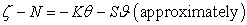(1)

Introducing the values of N, K and S into equation (1) reduces it to;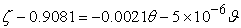(2)(3)

Where

(θ) = Latitude angle of the location of the solar collector (deg.)

(ϑ) = Exposure time (days)

(ζ ) = Transmissivity

K = 0.0021, N = 0.9081 and S = 5 x 10-6. N, K and S are empirical constants (determined using C-NIKBRAN 

### 4. Boundary and Initial Condition

Consider solar radiations incident to the solar collector positioned at different latitude angles. The amount of incident radiations on the collector is slightly affected by gases, water vapour and dusts in the atmosphere. Range of latitude angles and exposure time of solar collector considered are 12.34-13.06 deg. and 59-365 days respectively. The environmental weather condition, sun’s radiation on the collector location and the duration of the radiant inflow, the time of the day at which the sun’s radiant heat is being collected, the angle of incidence (i.e the angle between the normal to the solar array and a light ray from the sun), the solar distance (i.e the distance of the array from the sun), the light transmission losses in cover glasses, cover adhesive and other optical elements and other process conditions are as stated in the experimental technique .

The boundary conditions are: All around the collector, a zero gradient for the gas scalar are assumed. Also zero gradients are assumed dusts and water vapour in the atmosphere. The solar collector is stationary during the incidence of the radiation except when moved to a new location (different latitude angles). The sides of the collector are taken to be symmetries.

### 5. Results and Discussion

The derived model is equation (3). The computational analysis of Table 1 gave rise to Table 2

#### Table 2. Variation ζ - 0.9081 with - 0.0021θ - 5 x 10-6ϑ

5.1. Model Validation

The validity of the model was found to be rooted on equation (2) where both sides of the equation are correspondingly approximately equal. Table 2 also agrees with equation (2) following the values of ζ - 0.9081 and - 0.0021θ - 5 x 10-6 ϑ evaluated from the experimental results in Table 1. In addition, the derived model was validated by comparing the transmissivity predicted by the model and that obtained from the experiment . This was done using the 4th Degree Model Validity Test Techniques (4th DMVTT); computational, graphical, statistical and deviational analysis 

5.2. Statistical Analysis
5.2.1. Standard Error (STEYX)

The standard errors incurred in predicting transmissivity for each value of the latitude angle and solar collector exposure time considered as obtained from experiment and derived model were 0.0002 & 0.0003% and 0.0005 & 0.0001 % respectively. The standard error was evaluated using Microsoft Excel version 2003.

Figure 1. Coefficient of determination between transmissivity and latitude angle as obtained from experiment 

5.2.2. Correlation (CORREL)

The correlation coefficient between transmissivity and latitude angle & exposure time were evaluated from the results of derived model and experiment, considering the coefficient of determination R2 from Figure 1-Figure 4, was calculated using Microsoft Excel version 2003.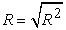(4)

The evaluated correlations are shown in Table 3. These evaluated results indicate that the derived model predictions are significantly reliable and hence valid considering its proximate agreement with results from actual experiment.

#### Table 3. Comparison of the correlations evaluated from derived model predicted and ExD results based on latitude angle and exposure time

5.3. Graphical Analysis

Comparative graphical analysis of Figure 5 and Figure 6 shows very close alignment of the curves from the experimental (ExD) and model-predicted (MoD) transmissivities. Furthermore, the degree of alignment of these curves is indicative of the proximate agreement between both experimental and model-predicted transmissivities.

5.4. Comparison of Derived Model with Standard Model
Figure 7. Comparison of transmissivites (relative to latitude angle as obtained from experiment , derived model and regression model
Figure 8. Comparison of transmissivites (relative to exposure time as obtained from experiment , derived model and regression model

The validity of the derived model was further verified through application of the regression model (Reg) (Least Square Method using Excel version 2003) in predicting the trend of the experimental results. Comparative analysis of Figs. 7 and 8 shows very close alignment of curves and significantly similar trend of data point’s distribution for experimental (ExD), derived model (MoD) and regression model-predicted (ReG) results of transmissivity. Also, the calculated correlations (from Figure 7 and Figure 8) between transmissivity and latitude angle & solar collector exposure time for results obtained from regression model gives 0.9998 & 0.9492 respectively. These values are in proximate agreement with both experimental and derived model-predicted results. The standard errors incurred in predicting transmissivity for each value of the latitude angle and solar collector exposure time considered as obtained from regression model were 2.8396 x10-5 and 2.1422 x 10-5 % respectively.

5.5. Computational Analysis

Comparative computational analysis of the experimental and model-predicted transmissivity was carried out to ascertain the degree of validity of the derived model. This was done by comparing results of evaluated transmissivities per unit latitude angle and exposure time resulting from solar radiation on the solar collector within latitude angle and exposure time ranges: 12.34-13.06 deg. and 59-365 days respectively as obtained from experimental and derived model.

5.5.1. Transmissivity Per Unit Latitude Angle

Transmissivity per unit latitude angle ζ / θ (deg.-1) was calculated from the equation;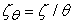(5)

Re-written as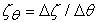(6)

Equation (6) is detailed as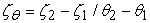(7)

Where

Δζ = Change in the transmissivity ζ 2, ζ 1 at two latitude angle values θ2 - θ1.

Considering the points (12.34, 0.8821) & (13.06, 0.8792), (12.34, 0.8819) & (13.06, 0.879) and (12.34,0.882) & (13.06, 0.879) as shown in Fig. 7, and designating them as (ζ1, θ1) & (ζ2, θ2) for experimental, derived model and regression model predicted results respectively, and then substituting them into equation (7), gives the slopes: - 0.0040, - 0.0040 and - 0.0042 deg-1 respectively as their corresponding transmissivity per unit latitude angle.

5.5.2. Transmissivity Per Unit Exposure Time

Similarly, substituting into equation (7) points (12.34, 0.8821) & (13.06, 0.8792), (12.34, 0.8819) & (13.06, 0.879) and (12.34, 0.882) & (13.06, 0.879) from Fig. 8, as (ζ1, ϑ1) & (ζ2, ϑ2) for experimental, derived model and regression model predicted results respectively also gives the slopes: - 1.0545 x 10-5, - 1.0545 x 10-5 and - 1.0909 x 10-5 day-1 respectively as their corresponding transmissivity per unit exposure time. The proximity between values in each result set indicates significantly high validity level for the derived model.

It is important to state that the actual transmissivity per unit exposure time and latitude angle (as obtained from experiment, derived model and regression model) is just the magnitude of the signed value. The associated sign preceding these values as evaluated, signifies that the associated slope tilted to negative plane. Based on the foregoing, the transmissivity per unit exposure time of the collector and latitude angle of its location as obtained from experiment, derived model & regression model were 1.0545 x 10-5, 1.0545 x 10-5 & 1.0909 x 10-5 (day)-1 and 0.0040, 0.0040 & 0.0042 deg.-1 respectively.

5.6. Deviational Analysis

A critical comparative analysis of the transmissivity values obtained from experiment  and derived model shows insignificant deviations on the part of the model-predicted values relative to values obtained from the experiment. This is attributed to the fact that the surface properties of the glazing cover material and the physiochemical interactions between the glazing material and gas molecules, water vapour as well as dust (under the influence of the temperature of the sun) which were believed to have played vital roles during the process  were not considered during the model formulation. This necessitated the introduction of correction factor,to bring the model-predicted transmissivity to those of the corresponding experimental values.

The deviation Dv, of model-predicted transmissivity from the corresponding experimental result is given by(8)

Correction factor, Cf to the model-predicted results is given by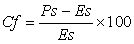(9)

Where

Es and Ps are transmissivities evaluated from experiment and derived model respectively.

Equations (8) and (9) indicate that correction factor is the negative of the deviation.

The correction factor took care of the negligence of operational contributions of surface properties of the glazing cover material and the physiochemical interactions between the glazing material and gas molecules, water vapour as well as dust (under the influence of the temperature of the sun) which actually played vital role during the solar radiation. The model predicted results deviated from those of the experiment because these contributions were not considered during the model formulation. Introduction of the corresponding values of Cf from equation (9) into the model gives exactly the corresponding experimental transmissivity.

#### Table 4. Deviation of model-predicted transmissivity (from that of experiment)

Table 4 show that the maximum deviation of the mode-predicted transmissivity from the corresponding experimental values is less than 0.03% and quite within the acceptable deviation limit of experimental results.

Consideration of equation (8) and critical analysis of Table 4 and Figure 1 - Figure 6 show that the least and highest magnitudes of deviation of the model-predicted transmissivity (from the corresponding experimental values) are - 0 and - 0.03%. This corresponds to transmissivity: 0.8790 and 0.8813; latitude angles: 13.01 and 12.46 deg. as well as solar collector exposure times: 365 and 120 days respectively.

On the other hand, consideration of equation (9) and critical analysis of Table 4 also indicates that the evaluated correction factors are negative of the deviation as shown in equations (8) and (9). Table 4 and Figs. 1- 6 also show that the least and highest correction factor (to the model-predicted transmissivity) are + 0 and + 0.003% which correspond to transmissivity: 0.8790 and 0.8813; latitude angles: 13.01 and 12.46 deg. as well as solar collector exposure times: 365 and 120 days respectively.

It is important to state that the deviation of model predicted results from that of the experiment is just the magnitude of the value. The associated sign preceding the value signifies that the deviation is a deficit (negative sign) or surplus (positive sign).

### 6. Conclusion

The factorial analysis of energy transmissivity by solar collector based on the collector exposure time and latitude angle of its location has been carried out. The two-factorial model derived and validated was used for the predictive analysis. The model structure highlighted the dependency of solar energy transmissivity on the collector exposure time and latitude angle of its location. The validity of the derived model was rooted on the core model expression ζ - 0.9081 = - 0.0021θ - 5 x 10-6 ϑ where both sides of the expression are correspondingly approximately equal. Evaluations from generated results indicated that transmissivity per unit exposure time of collector and latitude angle of its location as obtained from experiment, derived model & regression model were -1.0545 x 10-5, - 1.0545 x 10-5 & 1.0909 x 10-5 (day)-1 and - 0.0040, -.0040 & 0.0042 deg.-1 respectively. Standard errors incurred in predicting transmissivity for each value of the solar collector exposure time & latitude angle considered as obtained from experiment, derived model & regression model were 0.0003, 0.0002 & 2.1422 x 10-5 % and 0.0001, 0.0005 & 2.8396 x10-5 % respectively. The maximum deviation of model-predicted transmissivity (from experimental results) was less than 0.03% which is insignificant. This implies a model operational confidence level above 99.9%.

### References

  Far Ultraviolet Spectroscopic Explorer, FUSE: Space Based Astronomy, FACT Sheet, NASA, (1999).In article  Agbaka, A. C. (1996).Solar Power and Application.Celbez Publishers, Owerri, Nigeria.In article  Tiwari, G. N. and Suneja, S. (2002). Solar Thermal Engineering System. New Delhi. Arosa Publishing House, pp. 3.In article  Sukhatme, S. P. (1984). Solar Energy: Principles of Thermal Collection and Storage. Tata McGraw-Hill Publishing Company Limited, New Delhi.In article PubMed  Bello, Y. I., and Dillip, K. D. (2011). Transmissivity of the Glazing Surface of a Solar Flat Plate Collector Based on the Metrological Parameters of Yola, Nigeria. Journal of American Science. 7(1):639-643.In article  Garg, H. P. and Prakash, J. (2000). Solar Energy Fundamentals and Application. New Delhi: Tata McGraw-Hill.In article  Nwoye, C. I., (2008). Data Analytical Memory; C-NIKBRAN.In article PubMed  Nwoye, C. I., and Nwabanne J. T. (2013). Empirical Analysis of Methane Gas Yield Dependence on Organic Loading Rate during Microbial Treatment of Fruit Wastes in Digester. Advances in Applied Science Research 4(1):308-318.In article